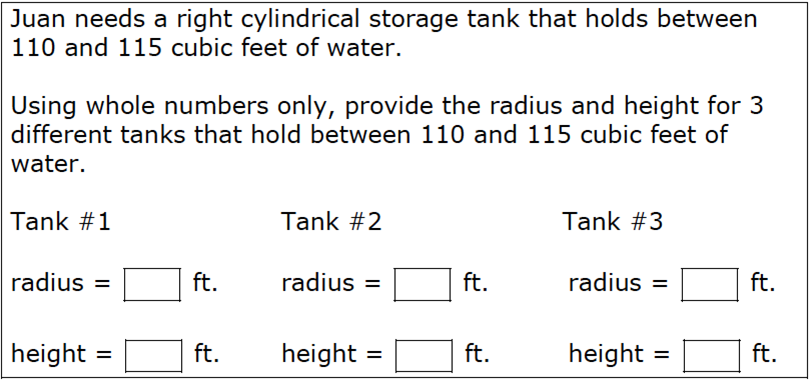# Cylinders### Hint

Is there a formula that can help us solve this problem?

When it says, “the tank holds between 110 and 115 cubic feet of water,” what mathematical concept are they referring to?

### Answer

r=1 and h = 36, r = 2 and h = 9, r = 3 and h = 4, r = 6 and h = 1

## Triangle Sum Theorem

Directions: Using the digits 1-9 at most one time each, fill in the blanks so …

### 8 comments

1.Also, (1 , 36 )= (r , h )

•Thanks Dan. I added your answer to the list.

2.3.I also like this question, but not as a standardized test item. These types of questions are great for classroom discussion. Their beauty is lost when the context is testing.